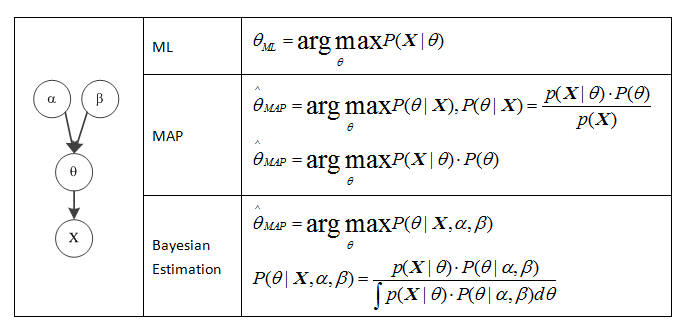• 参数估计的三种方法 机器学习有三种典型的参数估计方法，分别是极大似然估计(MLE)，极大后验估计(MAP)以及贝叶斯估计。除了参数估计，每种方法还对应有样本的密度估计。假设X=[xi]i=1nX=[x_i]_{i=1}^nX=[xi​]i=1n​...
参数估计的三种方法
机器学习有三种典型的参数估计方法，分别是极大似然估计(MLE)，极大后验估计(MAP)以及贝叶斯估计。除了参数估计，每种方法还对应有样本的密度估计。假设$X=[x_i]_{i=1}^n$。
MLE
极大似然是通过下式估计参数。
$\theta^* = argmax_\theta P(X|\theta)$
该式可以这样理解，在给定参数$\theta$的情况下，求解具体概率$P(X|\theta)$的最大值，使得满足预期样本分布。上式往往通过下述方法求解：
$\theta^* = argmax_\theta P(X|\theta)=argmax_\theta \prod_{i=1}^nP(x_i|\theta)$
MAP
极大后验则给定样本的后验和参数的先验，求解下式：
$\theta^* = argmax_\theta P(\theta|X)$
可以这样理解，在给定观测样本集的条件下，估计最有可能的$\theta^*$。该式往往利用Bayes公式求解，由于：
$P(\theta|X) = \frac{P(X|\theta)P(\theta)}{P(X)}$
所以：
$\theta^* = argmax_\theta P(\theta|X) = argmax_\theta \frac{P(X|\theta)P(\theta)}{P(X)} = argmax_\theta \prod_{i=1}^n P(x_i|\theta)P(\theta)$
其中，$P(\theta)$为参数的先验。
贝叶斯估计
MLE和MAP方法有着共同点，即他们都是估计最可能的$\theta$，并极大化上述式子求解$\theta$，这被称为点估计。而Bayes估计承认明显的事实，参数$\theta$可能有多个与数据兼容的值，用Bayes公式计算在数据X上参数$\theta$的概率分布。
$P(\theta|X) = \frac{P(X|\theta)P(\theta)}{P(X)} = \frac{\prod_{i=1}^n P(x_i|\theta)P(\theta)}{P(X)}$
注意，尽管和MAP形式上一致，但贝叶斯估计无极大化过程。且有：
$P(\theta|X)P(X) = P(X|\theta)P(\theta)$
$\int P(\theta|X)P(X) d\theta = \int P(X|\theta)P(\theta) d\theta = P(X)$
密度估计
样本的密度估计即估算预测分布。即计算下式：
$P(x^*|X) = \int P(x^*|\theta)P(\theta|X)d\theta$
可以这样理解，$P(x^*|\theta)$是一个给定值$\theta$的预测。所以，积分可以当做由不同参数$\theta​$确定的预测的加权和，也就是期望。
由于MLE和MAP这两种参数估计方法均属于点估计，因此这两者在进行预测时
$P(x^*|X) = \int P(x^*|\theta)\delta(\theta-\theta^*)d\theta$
其中$\delta(z)$积分为1，并且在除了z=0以外的任何点都为0。这是因为，在点估计方式下，隐含的假设为：$\theta$只有一个可能的最优值$\theta^*$。因此这两种方法下，有：
$P(\theta^*|X) = \infty$
且$P(\theta \neq \theta^*|X) = 0$
由于$P(x^*|X) = \int P(x^*|\theta)\delta(\theta-\theta^*)d\theta = P(x^*|\theta^*)$。
因此，MLE和MAP的密度估计方法自然导出：给定$\theta^*$，预测样本的分布。
贝叶斯估计中却不是这种方法，这是因为贝叶斯估计给出的$P(\theta|X)$是概率分布，非极大化的结果。所以，无法用$\delta$函数对$P(\theta|X)$进行替换。
举例-一元正态分布
假设n个数据点$[x_i]_i=1^n$从均值方差均为1的正态分布中采样得到。本例的目的是利用这些观测数据重新估算参数$\mu, \sigma^2$。
MLE
利用极大似然估计，我们有
$P(X|\mu, \sigma^2) = \prod_{i=1}^n P(x_i|\mu, \sigma) = \prod_{i=1}^n Norm_{x_i}(\mu,\sigma)$
其中$Norm_{x_i}(\mu,\sigma) = \frac{1}{\sqrt{2\pi \sigma}}e^{-\frac{(x_i-\mu)^2}{\sigma^2}}$为点$x_i$的概率密度。
极大似然估计即：
$\mu^*,\sigma^{2*} = argmax_{\mu, \sigma^2} P(X|\mu,\sigma) = argmax_{\mu, \sigma^2} log P(X|\mu,\sigma^2) ​$
对$P(X|\mu,\sigma^2)$取对数，然后对$\mu,\sigma^2$分别求偏导为0可得最优解。
MAP
利用极大后验估计，我们有
$P(\mu,\sigma^2|X) = \frac{P(X|\mu,\sigma^2)P(\mu,\sigma^2)}{P(X)}$
其中$P(X|\mu,\sigma^2)$和MLE中一致，$\mu,\sigma^2$的先验分布假设为正态逆伽马分布。表达式如下：
$P(\mu,\sigma^2)$
$= NormInvGamma_{\mu,\sigma^2}(\alpha,\beta,\gamma,\delta)$
$= \frac{\sqrt{\gamma}}{\sigma\sqrt{2\pi}} \frac{\beta^\alpha}{\Gamma(\alpha)}(\frac{1}{\sigma^2})^{\alpha+1} e^{-\frac{2\beta+\gamma(\delta-\mu)^2}{2\sigma^2}}$
正态逆伽马分布是正态分布的共轭，它有4个参数,分别是$\alpha,\beta,\gamma,\delta$。共轭性具有以下特点：
任意分布与其共轭分布乘积的结果正比于一个具有新参数的共轭分布。我们以正态分布和正态逆伽马分布为例：
$Norm_{x_i}(\mu,\sigma^2)*NormInvGamma_{\mu,\sigma^2}(\alpha,\beta,\gamma,\delta) = \chi(x_i, \alpha,\beta,\gamma,\delta) * NormInvGamma_{\mu,\sigma^2}(\hat{\alpha}, \hat{\beta}, \hat{\gamma}, \hat{\delta})$
这在贝叶斯估计中很有用，具体地我们会在下一小节讲述。
回到正题，现在我们得到了$P(\mu,\sigma^2|X)$，对其直接进行极大化，通过对参数求偏导为0可求解得到最优解。
$\mu^*,\sigma^{2*}= argmax_{\mu,\sigma}P(\mu,\sigma^2|X) = argmax_{\mu,\sigma}log P(\mu,\sigma^2|X)$
贝叶斯估计
贝叶斯估计则要复杂一些，它和MAP具有同样的形式，不同的是在最后一步。
$P(\mu,\sigma^2|X)​$
$= \frac{P(X|\mu,\sigma^2)P(\mu,\sigma^2)}{P(X)}​$
$= \frac{\prod_{i=1}^n Norm_{x_i}(\mu,\sigma^2)NormInvGamma_{\mu,\sigma^2}(\alpha,\beta,\gamma,\delta)}{P(X)}​$
$= \frac{NormInvGamma_{\mu,\sigma^2}(\hat{\alpha}, \hat{\beta}, \hat{\gamma}, \hat{\delta})\prod_{i=1}^n \chi(x_i, \alpha,\beta,\gamma,\delta) }{P(X)}​$
由于后验$P(\mu,\sigma^2|X)$必须是有效的概率分布且和为1，而$NormInvGamma_{\mu,\sigma^2}(\hat{\alpha}, \hat{\beta}, \hat{\gamma}, \hat{\delta})$也是一个概率分布且和为1。因此有：
$P(\mu,\sigma^2|X)=NormInvGamma_{\mu,\sigma^2}(\hat{\alpha}, \hat{\beta}, \hat{\gamma}, \hat{\delta})$
其中，$\hat{\alpha} = \alpha+n/2, \hat{\gamma}=\gamma+n, \hat{\delta} = \frac{\gamma\delta+\sum x_i}{\gamma+n}, \hat{\beta} = 0.5\sum x_i^2 + \beta+0.5\gamma\delta^2-\frac{(\gamma\delta+\sum x_i)^2}{2(\gamma+n)}$
可以看到，利用贝叶斯公式我们可以得到参数的概率分布，这里我们看到使用共轭先验进行贝叶斯估计的第一个优点，比起MLE和MAP，这种方法得到的结果是一个closed-solution。并且，参数概率分布的峰值即为MAP的解。
当数据比较多的时候，参数被指定好，概率分布比较集中，此时把所有概率量放到MAP估计处是对后验的一个好的逼近。然而，当数据稀少的时候，许多可能的参数也许已经解释了数据并且后验很宽，这种情况下用点估计方法是不合适的。
密度估计
对于MLE和MAP，由于它们是点估计。可以分别直接采用$P(x^*|\mu^*,\sigma^{2*})$和$P(x^*|\mu^*,\sigma^{2*})P(\mu^*,\sigma^{2*})$预测新样本$x^*$的密度。
对于贝叶斯估计方法，有：
$P(x^*|X)$
$= \int \int P(x^*|\mu,\sigma^2)P(\mu,\sigma^2|X)d\mu d\sigma$
$=\int\int Norm_{x^*}(\mu,\sigma^2)NormInvGamma_{\mu,\sigma^2}(\hat{\alpha}, \hat{\beta}, \hat{\gamma}, \hat{\delta})d\mu d\sigma$
$=\chi(x^*,\hat{\alpha}, \hat{\beta}, \hat{\gamma}, \hat{\delta}) \int \int NormInvGamma_{\mu,\sigma^2}(\overline{\alpha}, \overline{\beta}, \overline{\gamma}, \overline{\delta})d\mu d\sigma$
显然，积分项为1，所以有：
$P(x^*|X) =\chi(x^*,\hat{\alpha}, \hat{\beta}, \hat{\gamma}, \hat{\delta})=\frac{1}{\sqrt{2\pi}}\frac{\sqrt{\hat{\gamma}}}{\sqrt{\overline{\gamma}}}\frac{\hat{\beta^{\hat{\alpha}}}}{\overline{\beta}^{\overline{\alpha}}}\frac{\Gamma(\overline{\alpha})}{\Gamma(\hat{\alpha})}$
其中，
$\overline{\alpha} = \hat{\alpha}+n/2, \overline{\gamma}=\hat{\gamma}+n, \overline{\delta} = \frac{\hat{\gamma}\hat{\delta}+\sum x_i}{\hat{\gamma}+n}, \overline{\beta} = 0.5\sum x_i^2 +\hat{ \beta}+0.5\hat{\gamma}\hat{\delta}^2-\frac{(\hat{\gamma}\hat{\delta}+\sum x_i)^2}{2(\hat{\gamma}+n)}$。
这里，我们可以看到使用共轭先验进行贝叶斯估计的第二个优点，它意味着积分是可以计算的，所以可以得到密度预测的一个不错的闭式表达式。
对大量数据而言，贝叶斯估计和MAP估计得到的样本密度是非常接近的。但是在样本量少的情况下，贝叶斯估计的分布更加平稳，这也意味着它更不确定样本密度的真实性。


展开全文MAP
• 常见的三种参数估计的方法：最大似然估计法、最大后验估计法、贝叶斯估计法 示例情景：θ为抛一次硬币下面朝上的概率，其服从参数α和β的Beta分布，而X=(x1, x2)则是一个向量，表示观测结果，x1表示观测到正面的...
常见的三种参数估计的方法：最大似然估计法、最大后验估计法、贝叶斯估计法
示例情景：θ为抛一次硬币下面朝上的概率，其服从参数α和β的Beta分布，而X=(x1, x2)则是一个向量，表示观测结果，x1表示观测到正面的个数，x2表示观测到反面的个数。
其中α、β、X为已知量，我们的目标是求参数θ
ML：最大似然估计法
MAP：最大后验估计法
Bayesian Estimation：贝叶斯估计法展开全文贝叶斯 先验概率
• 主要是关于logistic的三种参数的算法：Yule算法，Rhodes算法，Nair算法matlab算法的实现。
• DeepLearningBook----reading notes 5.4 Estimators, Bias, and Variance 对于机器学习而言，parameter ...点估计尝试提供对于参数的最好预测。所谓的参数可以是参数化模型（parametric model）中单个参数
DeepLearningBook----reading notes
5.4 Estimators, Bias, and Variance
对于机器学习而言，parameter estimation，bias和variance是三个非常基础的概念，对于形式化地定义generalization、underfitting、overfitting而言非常重要。
5.4.1 Point Estimation
点估计尝试提供对于参数的最好预测。所谓的参数可以是参数化模型（parametric model）中的单个参数或者参数向量，比如线性回归中的权重$w$，同时也可以是整个函数$f$。
为了将参数的估计值和真值区分开，我们约定用$\hat{\theta}$来表示$\theta$的点估计（point estimation）。
$\{x_1, ...,x_m\}$是$m$个独立同分布（iid）的样本点集合，
点估计（point estimation or statistic）是指任意的关于数据的函数：
$\hat{\theta}_m=g(x^{(1)},...,x^{(m)})$
一个好的点估计器应该是这样的一个函数：这个函数的输出$\hat{\theta}_m$和真正的$\theta$足够接近。
到现在为止，我们采用的是统计学中频率学家的观点。即我们假设真实参数的值$\theta$是固定的但是未知的（fixed but unknown），同时点估计$\hat{\theta}$是关于数据（data）的函数。因为数据是从随机过程中（random process）抽样得到的，所以任何关于该数据的函数也是随机的（random），因此点估计$\hat{\theta}$也是一个随机变量（random variable）。
点估计也可以是关于输入和目标变量之间的关系的估计，我们称这种类型的点估计为函数估计器（function estimators）。
Function Estimation
当给定输入向量$\bold{x}$预测目标变量$\bold{y}$时，我们假定存在一个函数$f(\bold{x})$能够近似地描述$\bold{x}$和$\bold{y}$之间的关系。例如，我们可以假设$\bold{y}=f(\bold{x})+\epsilon$，其中$\epsilon$表示$\bold{y}$中不能从$\bold{x}$中预测的部分。在函数估计中，我们感兴趣的是用一个模型或者函数$\hat{f}$去近似真实的$f$。函数估计和参数$\theta$的点估计其实是一回事；函数估计器$\hat{f}$就是函数空间中的一个点估计器。线性回归和多项式回归既可以看做是参数$w$的点估计，也可以理解为$\bold{x}$和$\bold{y}$之间的一个函数估计器$\hat{f}$。
现在我们回顾一下点估计器的重要性质。
5.4.2 Bias
估计器的偏差定义为：
$bias(\hat{\theta}_m)=\bold{E}(\hat{\theta}_m)-\theta$
其中，我们是对整个数据集取期望，$\theta$是真实参数的值。如果$bias(\hat{\theta}_m)=0$，我们说估计器$\hat{\theta}_m$是无偏估计器（unbiased）,意味着$\bold{E}(\hat{\theta}_m)=\theta$。我们说估计器$\hat{\theta}_m$是渐进无偏的（asymptotically unbiased）如果$\begin{matrix} \lim_{m \to \infty}bias(\hat{\theta}_m)=0\end{matrix}$，即$\begin{matrix} \lim_{m \to \infty}\bold{E}(\hat{\theta}_m)=\theta\end{matrix}$。
书中主要举了两个例子来说明这里的概念，一个是Bernoulli Distribution，一个是Gaussian Distribution，后续有空在补上这两个例子的具体内容。
这些例子主要是说明：我们能够得到关于一个变量的两个估计器，一个是unbiased，一个是biased；尽管无偏估计器是我们想要的，但是在某些情况下无偏估计器可能并不是最好的估计器；在很多情况下我们都会使用有偏估计器，因为有偏估计器具有一些良好的性质（具备什么样的性质？）
5.4.3 Variance and Standard Error
后续有时间在补充完善。这里先记录一下几个重要的点。
对于一个估计器而言，我们想要这个估计器同时具有low bias和low variance（但是这个很难做到，涉及到trade off between bias and variance）。
在测试集上的样本的数量决定了这个估计器的准确程度。
估计器的方差会随着样本数量$m$的增加而减少，对于大部分的估计器而言，这个性质都是成立的。
5.5 Maximum Likelihood Estimation
前面的章节我们给出了估计器的定义以分析了估计器的相关性质如偏差和方差。但是这些估计器是怎么得到的呢？我们希望能够有一些准则，通过利用这些准则针对不同的模型能够推导出一些好的估计器。最常用的准则之一是maximum likelihood principle，即极大似然估计。
给定一个样本集合$\mathbb{X}=\{x^{(1)},...,x^{(m)}\}$，每个样本都是独立地从真实存在的但是未知的数据生成概率分布$p_{data}(\bold{x})$中生成的。令$p_{model}(\bold{x};\theta)$表示一类以$\theta$为参数的概率分布族，我们的目的是让$p_{model}(\bold{x};\theta)$尽可能地接近$p_{data}(\bold{x})$。
$\theta$的极大似然估计器可以表示为：
$\theta_{ML}=arg\,\max_{\theta}p_{model}(\mathbb{X};\theta)\\ =arg\,\max_{\theta}\prod_{i=1}^mp_{model}(x^{(i)};\theta)\\$
通常来说我们不希望优化目标表达式中存在连乘项，因为这样的表达式可能会出现数值溢出的问题。一般做法是对连乘项取对数，变成累加项，具体形式为：
$\theta_{ML}=arg\,\max_{\theta}\sum_{i=1}^m\log p_{model}(x^{(i)};\theta)$
对上述表达式做一个$\frac{1}{m}$的缩放，变成：
$\theta_{ML}=arg\,\max_{\theta}\bold{E}_{\bold{x}\sim\hat{p}_{data}}\log p_{model}(x;\theta)\tag{1}$
我们可以这样理解极大似然估计：极大似然估计等价于最小化由训练数据集定义的经验概率分布$\hat{p}_{data}$和由模型所定义的概率分布$p_{model}$之间的差异，而这种差异是由KL散度来衡量的。KL散度的定义为：
$D_{KL}(\hat{p}_{data}||p_{model})=\bold{E}_{\bold{x}\sim\hat{p}_{data}}[\log \hat{p}_{data}(x)-\log p_{model}(x)]\tag{2}$
观察一下表达式2，我们发现：
$\bold{E}_{\bold{x}\sim\hat{p}_{data}}\log \hat{p}_{data}(\bold{x})$
是给定的训练数据集的信息熵，是一个常数，于是表达式2等价于：
$min-\bold{E}_{\bold{x}\sim\hat{p}_{data}}\log p_{model}(\bold{x})\tag{3}$
可以看出，表达式1和表达式3的优化目标是相同的。
最小化KL散度等价于最小化不同分布间的交叉熵。许多研究人员用**“cross-entropy”**来特指Bernoulli或者softmax分布的负对数似然（negative log-likelihood,NLL），这其实是不对的；任何一种包含了NLL的损失函数都是由训练数据集所定义的经验分布和由模型所定义的概率分布之间的交叉熵损失函数。比如，MSE是经验分布和高斯模型之间的交叉熵（这里不懂）。
极大似然估计的目的是让由模型所定义的概率分布$p_{model}(\bold{x};\theta)$尽可能接近经验概率分布$\hat{p}_{data}(\bold{x};\theta)$；理想情况下，我们希望让$p_{model}(\bold{x};\theta)$逼近$p_{data}(\bold{x})$，但是我们没有办法获取到概率分布$p_{data}(\bold{x})$的任何信息。
我们可以通过优化极大似然函数或者最小化KL散度来得到参数$\theta$的估计值，通常把目标函数称为损失函数（cost function）。极大似然估计等价于最小化NLL，等价于最小化KL散度，等价于最小化交叉熵损失函数。
5.5.2 Properties of Maximum Likelihood
当训练样本数量$m\rightarrow\infty$，极大似然估计器被证明是渐进最优估计器（the best estimator asymptotically）。在合适的条件下，极大似然估计器有consistency的性质（后续在补充），即随着训练样本的数量趋近于无穷时，极大似然估计器得到的参数收敛于参数真值。这些条件是：

真实概率分布$p_{data}$必须和模型概率分布$p_{model}(\bold{x};\theta)$是同一类概率分布；（不确定是否翻译正确，原文为：The true distribution $p_{data}$ must lie within the model family $p_{model}$）不然，没有一个估计器能够恢复$p_{data}$；（感觉这个条件已经很强了，有点像共轭的性质？）
真实的概率分布$p_{data}$必须和参数$\theta$的某一个值相同。不然，即使极大似然估计器能够恢复$p_{data}$，但是也不能确定用来产生数据的$\theta$是哪一种具体取值。

除了极大似然估计准则外，还有很多其他很好的准则，这些准则大多数都是consistent estimators。但是不同的估计器之间他们的统计效率（statistic efficiency）是不同的，比如在固定的训练样本数量下，有些估计器能够取得更低的泛化误差，在取得固定的泛化误差前提下，有些估计器所需要的样本数量更少。
Cramer-Rao lower bound表明没有任何一个consistent估计器的MSE比极大似然估计器低。
考虑到consistency和统计效率的原因，在机器学习中我们通常采用极大似然估计。当少量的样本已经产生过拟合现象时，我们会对极大似然估计添加正则项比如权重衰减（weight decay，所谓的L2正则）以便于在训练样本比较少的情况下我们的估计器还能够获得较小的方差。
5.6 Bayesian Statistics
目前为止我们讨论的是频率学派统计学（frequentist statistics）和基于点估计器的方法。另外一种统计学流派是贝叶斯统计学（Bayesian statistics），主要思想是在做预测的时候考虑$\theta$的所有取值可能。
频率学派认为真实参数值$\theta$是固定的但是未知的，并且点估计$\hat{\theta}$是一个随机变量，因为$\hat{\theta}$是关于数据集的函数，并且数据集也被视为随机变量。贝叶斯使用概率来表示我们对信息的确信度。数据集是被直接观测的，所以不是随机的。也就是说真实参数$\theta$是未知的、不确定的，因此$\theta$应该被视为随机变量。从两者的表达式也可以看出不同；频率学派的表达式一般为：$p(x;\theta)$，贝叶斯学派的表达式一般为：$p(x,\theta)$，前者的表达式$\theta$不是一个随机变量，后者的表达式$\theta$是随机变量。
在观测到数据前，我们会使用先验概率分布（prior probability distribution，有时记做"the prior"），$p(\theta)$来反映参数$\theta$的不确定程度。通常来说，实践人员会选择具有大信息熵的概率分布作为先验分布以此反映参数的高不确定性。比如我们可能假设先验分布属于均匀分布（具有很高的信息熵），倾向于选择更简单的解决方案（比如small magnitude coefficients（这也是L2正则的目的），接近于常量的函数）。
通过以下表达式我们能够得到关于参数$\theta$的后验分布：
$p(\theta|x^{(1)},...,x^{(m)})=\frac{p(x^{(1)},...,x^{(m)}|\theta)p(\theta)}{p(x^{(1)},...,x^{(m)})}$
通常来说我们会选择均匀分布或者高斯分布作为先验分布（具有高信息熵），观测到的数据会降低分布的信息熵并且让分布集中于具有高取值可能性的参数范围。
和极大似然估计相比，贝叶斯估计主要有两点不同：

极大似然估计是对$\theta$的点估计，贝叶斯估计利用a full distribution over $\theta$来做预测；比如，在观测到$m$个样本之后，对于下一个样本$x^{(m+1)}$的预测概率分布为：
$p(x^{(m+1)}|x^{(1)},...,x^{(m)})=\int p(x^{(m+1)}|\theta)p(\theta|x^{(1)},...,x^{(m)})\mathrm{d}\theta$
对于最终的预测而言，具有非零的概率密度的$\theta$值都有贡献，其权重为相应的后验概率密度。
频率学派通过评估估计器的方差来衡量一个点估计器的不确定性。贝叶斯估计是通过对估计器进行积分来处理估计器的不确定性，这样的方式能够比较好的防止过拟合（为什么？）。

第二个不同就是贝叶斯估计中多了贝叶斯先验概率分布；先验表达了我们更喜欢简单的、平滑的模型，同时也是人类对于事物的主观性认识和判断，从而去影响最终的预测。

当训练样本比较少的时候，贝叶斯估计的泛化能力更好，当训练样本很多的时候贝叶斯估计的计算代价很高（存在积分）。相比之下，训练样本少的时候，极大似然估计容易过拟合，当训练样本大的时候，极大似然估计具有很好的收敛性，同时计算代价并不高，这也是为什么神经网络的损失函数一般是用极大似然估计原理得到的，因为神经网络的数据量一般很大；这也是很多时候神经网络加weight decay的效果反而会变差（当然有时候加上会更好）。
5.6.1 Maximum A Posteriori(MAP) Estimation
贝叶斯估计是利用关于参数$\theta$的所有贝叶斯后验概率分布来做预测，但是这样的操作对于很多模型而言都是intractable；点估计提供了一个tractable的近似。和极大似然估计不同，我们仍然希望能够利用先验概率分布信息来影响点估计的选择。这就是所谓的maximum a posteriori（MAP），属于点估计，
$\theta_{MAP}=arg\,\max_{\theta}p(\theta|x)=arg\,\max_{\theta}\log p(x|\theta)+\log p(\theta)$
$arg\,\max_{\theta}\log p(x|\theta)$是标准的极大似然估计，$\log p(\theta)$是先验分布。
参数具有高斯先验分布的MAP贝叶斯推理等价于权重衰减（weight decay）的极大似然估计。
和全贝叶斯推理相比，MAP能够利用先验分布的信息，这样的信息能够减少MAP点估计器的方差（相比于ML），但是增加了偏差。
许多正则化的估计策略，比如极大似然估计，都能够被解释为贝叶斯推理的MAP近似。当正则化项包含$\log p(\theta)$时这样的解释都是成立的。当然，不是所有的正则化策略都可以理解为MAP，比如，不包含概率分布的对数的正则化项，比如依赖于具体数据的正则化项（先验分布是不依赖于具体数据的）。MAP能够让我们设计复杂但是可解释的正则化项，比如使用混合高斯分布推导得出的概率分布作为先验。
后续在补充其他知识点。


展开全文机器学习 深度学习
• 前几天上的机器学习课上，老师讲到了参数估计的三种方法：ML，MAP和Bayesian estimation。课后，又查了一些相关资料，以及老师推荐的LDA方面的论文《Parameter estimation for text analysis》。本文主要介绍文本...
    前几天上的机器学习课上，老师讲到了参数估计的三种方法：ML，MAP和Bayesian  estimation。课后，又查了一些相关资料，以及老师推荐的LDA方面的论文《Parameter estimation for text analysis》。本文主要介绍文本分析的三类参数估计方法-最大似然估计MLE、最大后验概率估计MAP及贝叶斯估计，以及三者之间的区别。

1、最大似然估计MLE

首先回顾一下贝叶斯公式

这个公式也称为逆概率公式，可以将后验概率转化为基于似然函数和先验概率的计算表达式，即

最大似然估计就是要用似然函数取到最大值时的参数值作为估计值，似然函数可以写做

由于有连乘运算，通常对似然函数取对数计算简便，即对数似然函数。最大似然估计问题可以写成

这是一个关于的函数，求解这个优化问题通常对求导，得到导数为0的极值点。该函数取得最大值是对应的的取值就是我们估计的模型参数。

以扔硬币的伯努利实验为例子，N次实验的结果服从二项分布，参数为P，即每次实验事件发生的概率，不妨设为是得到正面的概率。为了估计P，采用最大似然估计，似然函数可以写作

其中表示实验结果为i的次数。下面求似然函数的极值点，有

得到参数p的最大似然估计值为

可以看出二项分布中每次事件发的概率p就等于做N次独立重复随机试验中事件发生的概率。

如果我们做20次实验，出现正面12次，反面8次

那么根据最大似然估计得到参数值p为12/20 = 0.6。

2、最大后验估计MAP

最大后验估计与最大似然估计相似，不同点在于估计的函数中允许加入一个先验，也就是说此时不是要求似然函数最大，而是要求由贝叶斯公式计算出的整个后验概率最大，即

注意这里P（X）与参数无关，因此等价于要使分子最大。与最大似然估计相比，现在需要多加上一个先验分布概率的对数。在实际应用中，这个先验可以用来描述人们已经知道或者接受的普遍规律。例如在扔硬币的试验中，每次抛出正面发生的概率应该服从一个概率分布，这个概率在0.5处取得最大值，这个分布就是先验分布。先验分布的参数我们称为超参数(hyperparameter)即

同样的道理，当上述后验概率取得最大值时，我们就得到根据MAP估计出的参数值。给定观测到的样本数据，一个新的值发生的概率是

下面我们仍然以扔硬币的例子来说明，我们期望先验概率分布在0.5处取得最大值，我们可以选用Beta分布即

其中Beta函数展开是

当x为正整数时$\Gamma(n) = (n-1)!\,$

Beta分布的随机变量范围是[0,1],所以可以生成normalised probability values。下图给出了不同参数情况下的Beta分布的概率密度函数我们取$\Gamma(n) = (n-1)!\,$,这样先验分布在0.5处取得最大值，现在我们来求解MAP估计函数的极值点，同样对p求导数我们有$\Gamma(n) = (n-1)!\,$

得到参数p的的最大后验估计值为$\Gamma(n) = (n-1)!\,$

和最大似然估计的结果对比可以发现结果中多了$\Gamma(n) = (n-1)!\,$这样的pseudo-counts,这就是先验在起作用。并且超参数越大，为了改变先验分布传递的belief所需要的观察值就越多，此时对应的Beta函数越聚集，紧缩在其最大值两侧。

如果我们做20次实验，出现正面12次，反面8次，那么

那么根据MAP估计出来的参数p为16/28 = 0.571,小于最大似然估计得到的值0.6，这也显示了“硬币一般是两面均匀的”这一先验对参数估计的影响。

3 贝叶斯估计

贝叶斯估计是在MAP上做进一步拓展，此时不直接估计参数的值，而是允许参数服从一定概率分布。回顾一下贝叶斯公式$\Gamma(n) = (n-1)!\,$

现在不是要求后验概率最大，这样就需要求$\Gamma(n) = (n-1)!\,$,即观察到的evidence的概率，由全概率公式展开可得$\Gamma(n) = (n-1)!\,$

当新的数据被观察到时，后验概率可以自动随之调整。但是通常这个全概率的求法是贝叶斯估计比较有技巧性的地方。

那么如何用贝叶斯估计来做预测呢？如果我们想求一个新值$\Gamma(n) = (n-1)!\,$的概率，可以由$\Gamma(n) = (n-1)!\,$

来计算。注意此时第二项因子在$\Gamma(n) = (n-1)!\,$上的积分不再等于1，这就是和MLE及MAP很大的不同点。

我们仍然以扔硬币的伯努利实验为例来说明。和MAP中一样，我们假设先验分布为Beta分布，但是构造贝叶斯估计时，不是要求用后验最大时的参数来近似作为参数值，而是求满足Beta分布的参数p的期望，有注意这里用到了公式$\Gamma(n) = (n-1)!\,$

当T为二维的情形可以对Beta分布来应用；T为多维的情形可以对狄利克雷分布应用

根据结果可以知道，根据贝叶斯估计，参数p服从一个新的Beta分布。回忆一下，我们为p选取的先验分布是Beta分布，然后以p为参数的二项分布用贝叶斯估计得到的后验概率仍然服从Beta分布，由此我们说二项分布和Beta分布是共轭分布。在概率语言模型中，通常选取共轭分布作为先验，可以带来计算上的方便性。最典型的就是LDA中每个文档中词的Topic分布服从Multinomial分布，其先验选取共轭分布即Dirichlet分布；每个Topic下词的分布服从Multinomial分布，其先验也同样选取共轭分布即Dirichlet分布。

根据Beta分布的期望和方差计算公式，我们有可以看出此时估计的p的期望和MLE ，MAP中得到的估计值都不同，此时如果仍然是做20次实验，12次正面，8次反面，那么我们根据贝叶斯估计得到的p满足参数为12+5和8+5的Beta分布，其均值和方差分别是17/30=0.567, 17*13/(31*30^2)=0.0079。可以看到此时求出的p的期望比MLE和MAP得到的估计值都小，更加接近0.5。

综上所述我们可以可视化MLE,MAP和贝叶斯估计对参数的估计结果如下个人理解是，从MLE到MAP再到贝叶斯估计，对参数的表示越来越精确，得到的参数估计结果也越来越接近0.5这个先验概率，越来越能够反映基于样本的真实参数情况。

4.三者之间的区别

首先我们可以看到,最大似然估计和最大后验估计都是基于一个假设，即把待估计的参数π看做是一个固定的值，只是其取值未知。而最大似然是最简单的形式，其假定参数虽然未知，但是是确定值，就是找到使得样本对数似然分布最大的参数。而最大后验，只是优化函数为后验概率形式，多了一个先验概率项。
而贝叶斯估计和二者最大的不同在于，它假定参数是一个随机的变量，不是确定值。在样本分布P(π|χ)上，π是有可能取从0到1的任意一个值的，只是取到的概率不同。而MAP和MLE只取了整个概率分布P(π|χ)上的一个点，丢失了一些观察到的数据χ给予的信息（这也就是经典统计学派和贝叶斯学派最大的分歧所在。）

参考文献：
1.Gregor Heinrich, Parameter estimation for test analysis, technical report
2.文本语言模型的参数估计-最大似然估计、MAP及贝叶斯估计  http://blog.csdn.net/yangliuy/article/details/8296481
3.《Gibbs Sampling for the UniniTiated》阅读笔记(上)---参数估计方法及Gibbs Sampling简介 http://crescentmoon.info/2013/06/29/Gibbs%20Sampling%20for%20the%20UniniTiated-1/

展开全文机器学习
• 讨论了三种新颖策略，所有这些策略都是为了增强设计参数估计动态响应和快速收敛性能。 这些策略包括动态速度修改策略，基于免疫记忆搜索信息保存机制以及基于免疫网络PSO学习算子。最后，将一种提出方...研究论文
• ## 参数估计方法

千次阅读 2019-11-07 20:58:21
参数估计有多种方法，下面简单和大家分享以下两： 一、最大似然估计 原理： 最合理的参数估计量应该使得从模型中抽取该n组样本观测值概率最大，也就是概率分布函数或者说是似然函数最大。 二、最小二乘法 当从...
• 其次，当需要一新颖模糊LDA模型以对特征提取和分类做出某些决定时，模糊LDA模型动力学参数估计方法应在测量数据可用时对其进行递归处理。 按照先前观点，我们通过考虑称为HRF-LDAHopfield神经网络（HNN）...研究论文
• 机器学习中的参数估计方法 ... 前几天上的机器学习课上，老师讲到了参数估计的三种方法：ML，MAP和Bayesian estimation。课后，又查了一些相关资料，以及老师推荐的LDA方面的论文《Parameter estimation f
• 声明：本文总结是基于parameter estimation for text analysis这篇文章，想... 参数估计方法三种）：MLE、MAP和贝叶斯估计**两类常见Inference问题： Estimation问题：估计一个能够最好地描述观测值X分布贝叶斯 MAP Beta分布
• 1、参数估计三种方法 MLE和MAP是点估计，而第三种Bayesian方法则是求期望。 2、期望求解 其中： 这里面困惑点是分母这一全概率可否作为常数不参与计算。 如此，期望应等同于求解： 如此，用gibbs...
• 在分析自回归模型基础上,研究了Yule Walker,Burg和Covariance三种参数估计方法的检测原理,提出了一种改进Covariance方法。在Matlab仿真平台上,采用4种参数估计方法对谐波、间谐波、次谐波进行检测。仿真结果...
• 在这里，个古典 优化方法，例如遗传算法（GA），粒子 群优化（PSO）和模拟退火（SA）是 在系统模型上使用以优化密钥 ODE的参数。 之后，我们比较了他们优化 效果分别。 结果表明 PSO算法是优化关键参数的最佳...
• 在机器学习的参数估计（学习）中，常见三种更新方法：梯度下降，极大似然，EM算法；它们本质目的均是让模型更好地拟合目标分布；在学习三种算法联系前，需要先了解牛顿法； 牛顿法迭代 先从经典牛顿法开始，...算法 机器学习
• ARMA 序列递推预报例子 考虑下面 ARMA(4,2) 模型 利用 wold 系数以及自协方差函数公式 编程计算出前 21 个自协方差 加到无穷大可以用加到 50 来近似计算自协方差结果为利用上一讲得到公式计算 Yt 自协方差...c++求区间第k大数
• 第一篇随笔，打算把搞过的东西整理一下～test～ ...先来一发已发布的报告【Genetic Linkage Model】，主要介绍了利用Newton-Raphson、EM、Gibbs这三种经典算法对Genetic Linkage Model做参数估计的方法和R代码。 ...
• 对未知参数的估计方法三种：矩估计(运用p+q个样本自相关系数估计总体自相关系数)，极大似然估计(使得联合密度函数达到最大的参数值)，最小二乘估计(使得残差平方和达到最小那组参数值即为最小二乘估计)。...
• Matlab2016a实验内容：给定以下w1和w2的三维数据，考虑不同维数下的高斯概率密度模型：（a）编写程序，对表格中的类w1中的3个特征，分别求解最大似然估计。 （b）修改程序，处理二维数据的情形。然后处理对表格中的...机器学习 matlab 数据
• 以下以抛硬币为例比较三种参数估计方法。极大似然估计：根据样本概率分布，写出样本联合概率似然函数，通过最大化似然函数，得到参数估计值。只基于样本信息。步骤：（1）确定似然函数（2）将似然函数转换为对数...
• 一、抽样分布 （一）总体公布与总体参数 （二）统计量与抽样分布 1、统计量 统计量是样本函数。...抽取样本的方法： 重置抽样：抽取过程中总体单位总数始终不变 非重置抽样 样本均值抽样分布是指所
• 通过计算线性反问题部分稀疏解，实现了典范自回归分解（CARD）模型的参数估计。 通过构造一个超完备字典，可以证明正弦曲线解是稀疏，而有色噪声解则不是。 为了得出解决方案，提出了一交替优化算法，...
• 文章目录前言一、文章重点及流程梳理二、概率论基础知识参数估计1.极大似然估计（Maximum Likelihood Estimation）2.MAP（最大后验概率）3.两策略总结 前言 写作参考概率论书籍、西瓜书、李航《统计学习方法...机器学习
• 对于平稳随机信号，主要有三种常用线性模型：AR（Auto-Regression，自回归）模型、MA（Moving Average，滑动平均）模型和ARMA（Auto-Regression-Moving Average，自回归滑动平均）模型。 这里我们选定 AR 模型，用...
• 本次发布内容为“如何利用MATLAB程序跑出SAR、SEM和SDM三种空间计量模型的参数估计结果”，本文系范巧老师2019年5月18日-5月26日在东北师范大学经济学院培训部分内容。一、参考文献：范巧,Hudson Darren.一种新...
• 这里三篇文章就对三种参数估计方法进行简单介绍。 对一些数理统计基本概念介绍，可参考之前文章“数理统计学基本概念”。 参数点估计问题 设有一个统计总体，以f(x;θ1,⋯,θk)f(x;θ1,⋯,θk)f(x;\...数理统计
• 对未知参数的估计方法三种：矩估计(运用p+q个样本自相关系数估计总体自相关系数)，极大似然估计(使得联合密度函数达到最大的参数值)，最小二乘估计(使得残差平方和达到最小那组参数值即为最小二乘估计)。...
• 说明对未知参数的估计方法三种：矩估计（运用p+q个样本自相关系数估计总体自相关系数），极大似然估计（使得联合密度函数达到最大的参数值），最小二乘估计（使得残差平方和达到最小那组参数值即为最小二乘...时间序列 最小二乘估计 极大似然估计
• 并通过计算机仿真了这2信号谱相关平面图（即循环频率-频率双频维图），提出了利用循环谱相关函数截面图估计BPSK信号和QPSK信号调制参数（即载频和码元速率）并识别这2信号的方法. 搜索最大峰值可估计...
• 基于景象匹配制导的飞行器飞行前需要进行航迹规划, 就是在飞行区域中选择出一些匹配概率高的匹配...配概率, 并提出了基准图的三个特征参数, 最后通过线性分类器, 实现了用特征参数估计匹配概率的目标, 并进行了实验验证...